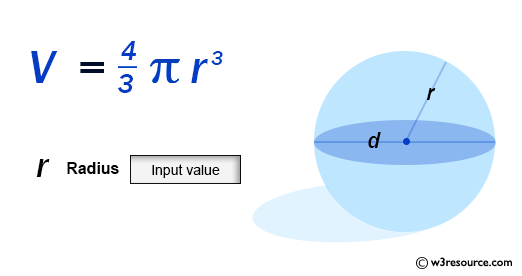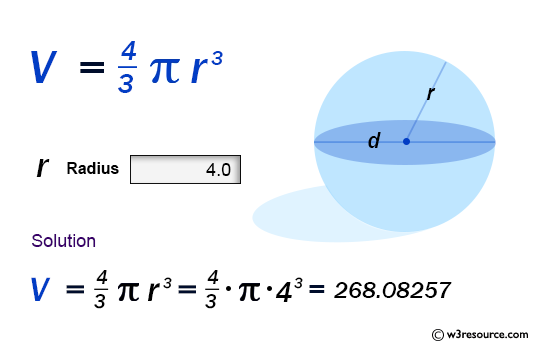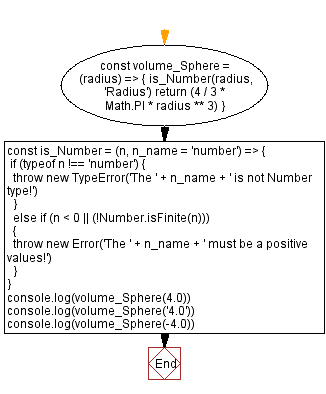# JavaScript: Volume of a Sphere

## JavaScript Math: Exercise-63 with Solution

Write a JavaScript program to calculate the volume of a Sphere.

From Wikipedia -
A sphere is a geometrical object that is a three-dimensional analogue to a two-dimensional circle. A sphere is the set of points that are all at the same distance r from a given point in three-dimensional space.That given point is the centre of the sphere, and r is the sphere's radius. The earliest known mentions of spheres appear in the work of the ancient Greek mathematicians.

Sample Data:Sample Solution:

HTML Code:

``````<!DOCTYPE html>
<html>
<meta charset="utf-8">
<title>JavaScript function to Volume of a Sphere</title>
<body>

</body>
</html>
```
```

JavaScript Code:

``````const volume_Sphere = (radius) => {
return (4 / 3 * Math.PI * radius ** 3)
}
const is_Number = (n, n_name = 'number') => {
if (typeof n !== 'number') {
throw new TypeError('The ' + n_name + ' is not Number type!')
}
else if (n < 0 || (!Number.isFinite(n)))
{
throw new Error('The ' + n_name + ' must be a positive values!')
}
}
console.log(volume_Sphere(4.0))
console.log(volume_Sphere('4.0'))
console.log(volume_Sphere(-4.0))
```
```

Sample Output:

```268.082573106329
----------------------------------------------------
Uncaught TypeError: The Radius is not Number type!
at https://cdpn.io/cpe/boomboom/pen.js?key=pen.js-19d5ed64-fc75-d66c-3f3c-fbac27f90859:7
---------------------------------------------------------------------------------------
Uncaught Error: The Radius must be a positive values!
at https://cdpn.io/cpe/boomboom/pen.js?key=pen.js-809901d5-f749-5312-6519-fd58b9f462c0:11
```

Flowchart:Live Demo:

See the Pen javascript-math-exercise-63 by w3resource (@w3resource) on CodePen.

Improve this sample solution and post your code through Disqus

Previous: Volume of a Pentagonal Prism.
Next: Volume of a Hemisphere

What is the difficulty level of this exercise?

Test your Programming skills with w3resource's quiz.

﻿